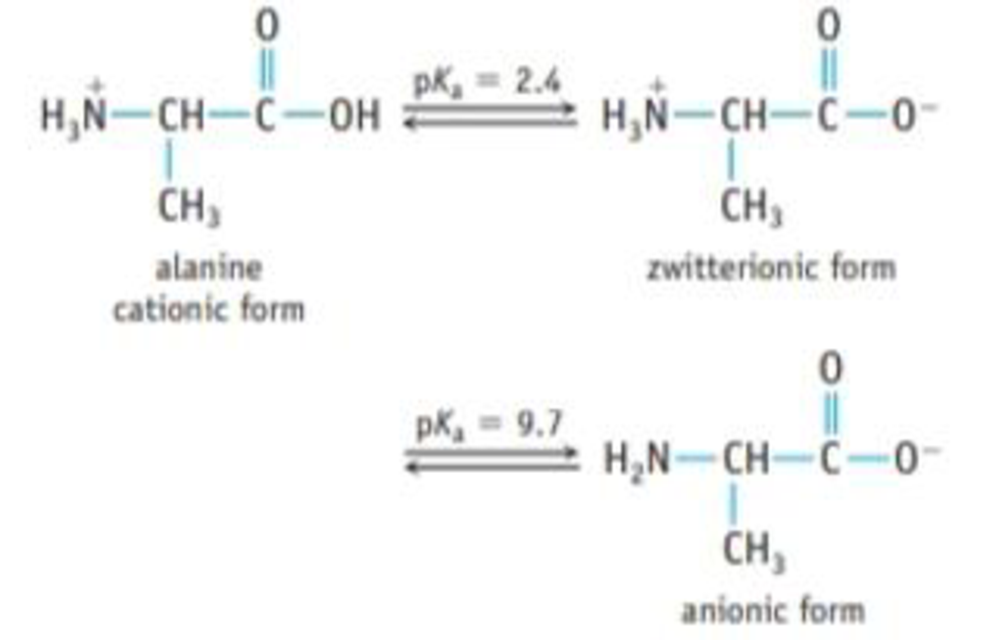# Amino acids are an important group of compounds. At low pH, both the carboxylic acid group (—CO 2 H) and the amine group (—NHR) are protonated. However, as the pH of the solution increases (say, by adding base), the carboxylic acid proton is removed, usually at a pH between 2 and 3. In a middle range of pHs, therefore, the amine group is protonated, but the carboxylic acid group has lost the proton. (This is called a zwitterion .) At more basic pH values, the amine proton is dissociated. What is the pH of a 0.20 M solution of alanine hydrochloride, [NH 3 CHCH 3 CO 2 H]Cl?### Chemistry & Chemical Reactivity

9th Edition
John C. Kotz + 3 others
Publisher: Cengage Learning
ISBN: 9781133949640

#### Solutions

Chapter
Section### Chemistry & Chemical Reactivity

9th Edition
John C. Kotz + 3 others
Publisher: Cengage Learning
ISBN: 9781133949640
Chapter 16, Problem 116IL
Textbook Problem
10 views

## Amino acids are an important group of compounds. At low pH, both the carboxylic acid group (—CO2H) and the amine group (—NHR) are protonated. However, as the pH of the solution increases (say, by adding base), the carboxylic acid proton is removed, usually at a pH between 2 and 3. In a middle range of pHs, therefore, the amine group is protonated, but the carboxylic acid group has lost the proton. (This is called a zwitterion.) At more basic pH values, the amine proton is dissociated.What is the pH of a 0.20 M solution of alanine hydrochloride, [NH3CHCH3CO2H]Cl?

Interpretation Introduction

Interpretation:

The pH has to be calculated for 0.20M solution of Alanine Hydrochloride.

Concept introduction:

An amino acid has both basic amine group (NH2) and an acid carboxylic acid (COOH) group.

The Henderson-Hasselbalch equation is,

pH=pKa+log([CONJUGATEBASE][ACID])

pH is a measure of hydrogen ion concentration and is calculated as:

pH=log[H+]

### Explanation of Solution

The pH of the solution of an Alanine is increases by adding a hydroxide ions, the hydrogen ion is removed from the NH3+ group.

CH3CH(NH3+)(COO)+OHCH3CH(NH2)(COO)+H2O

The pH of the solution of an alanine is decreases by adding an acid to a solution of alanine, the COO part of zwitter ion picks up a proton.

CH3CH(NH3+)(COO)+H+(aq)CH3CH(NH3+)(COOH)                  (3)

Given:

The concentration of Alanine Hydrochloride is ([COOH]+[COO])=0.20M    (4)

The pKa of carboxyl group of Alanine is 2.4.

The pKa of amine group of Alanine is 9.7.

The solution 0.20M Alanine HCl is composed of 0.20M Alanine and 0.20M HCl. Thus, there is an expectation to have an acidic pH and only the carboxyl group of Alanine is involved in proton equilibrium as in equation (3).

Substitute the value of acidic pKa in equation the below equation:

pH=pKa+log([CONJUGATEBASE][ACID])

pH=2

### Still sussing out bartleby?

Check out a sample textbook solution.

See a sample solution

#### The Solution to Your Study Problems

Bartleby provides explanations to thousands of textbook problems written by our experts, many with advanced degrees!

Get Started

Find more solutions based on key concepts
Diets with sufficient protein may provide more satiety than diets that are low in protein.

Nutrition: Concepts and Controversies - Standalone book (MindTap Course List)

29-8 How is glucose activated for glycogen synthesis?

Introduction to General, Organic and Biochemistry

What is meant by biological evolution?

Human Biology (MindTap Course List)

What is a codon?

Chemistry for Today: General, Organic, and Biochemistry

From what group are birds thought to have evolved?

Oceanography: An Invitation To Marine Science, Loose-leaf Versin

Which color light refracts the most when entering crown glass from air at some incident angle with respect to ...

Physics for Scientists and Engineers, Technology Update (No access codes included)